Function Repository Resource:

# KSetPartitions

Give all possible ways to partition a set into a given number of subsets, ignoring order of blocks and within blocks

Contributed by: Wolfram Staff
 ResourceFunction["KSetPartitions"][set,k] returns the list of set partitions of set with k blocks. ResourceFunction["KSetPartitions"][n,k] returns the list of set partitions of {1,2,…, n} with k blocks.

## Details and Options

A set partition of a set S is an unordered set of nonempty disjoint subsets of S (called blocks) whose union is S.
Both the order of the blocks and the order within each block are ignored.

## Examples

### Basic Examples (2)

There are 15 set partitions of a five-element set into two subsets:

 In:=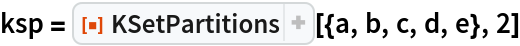Out=The number of such set partitions is given by a Stirling number of the second kind:

 In:=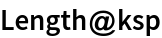Out=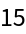In:=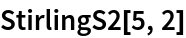Out=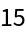### Scope (1)

Here is a compact way to see the blocks:

 In:=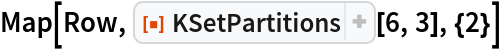Out=George Beck

## Requirements

Wolfram Language 11.3 (March 2018) or above

## Version History

• 1.0.0 – 11 March 2019